Courses

# Ex 13.5 NCERT Solutions- Surface Areas and Volumes Class 10 Notes | EduRev

## Class 10 : Ex 13.5 NCERT Solutions- Surface Areas and Volumes Class 10 Notes | EduRev

The document Ex 13.5 NCERT Solutions- Surface Areas and Volumes Class 10 Notes | EduRev is a part of the Class 10 Course Mathematics (Maths) Class 10.
All you need of Class 10 at this link: Class 10

NCERT TEXTBOOK QUESTIONS SOLVED
Page No. 258
EXERCISE 13.5

Q 1. A copper wire, 3 mm in diameter, is wound about a cylinder whose length is 12 cm, and diameter 10 cm, so as to cover the curved surface of the cylinder. Find the length and mass of the wire, assuming the density of copper to be 8.88 g per cm.
Sol. Since, diameter of the cylinder = 10 cm
∴ Radius of the cylinder (r) =  10/2 cm = 5 cm
⇒ Length of wire in completely one round
2πr = 2 × 3.14 × 5 cm = 31.4 cm
∵ Diameter of wire = 3 mm = 3/10 cm
∴ The thickness of cylinder covered in one round = 3/10 cm
⇒ Number of rounds (turns) of the wire to cover 12 cm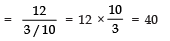∴ Length of wire required to cover the whole surface = Length of wire required to complete 40 rounds
= 40 × 31.4 cm = 1256 cm
Now, radius of the wire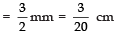∴ Volume of wire = πr2l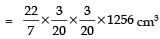∵ Density of wire = 8.88 gm/cm3
∴Weight of the wire = [Volume of the wire] × Density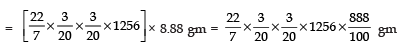= 788 g (approx.)

Q 2. A right triangle, whose sides are 3 cm and 4 cm (other than hypotenuse) is made to revolve about its hypotenuse. Find the volume and surface area of the double cone so formed. Choose value of π as found appropriate.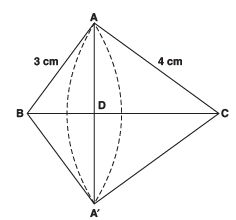Sol. Let us consider the rt ΔBAC, rt. angled at A such that
AB = 3 cm, AC = 4 cm
∴ Hypotenuse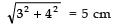Obviously, we have obtained two cones on the same base AA′ such that the radius = DA or DA'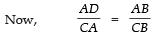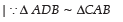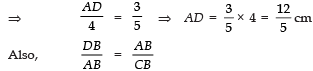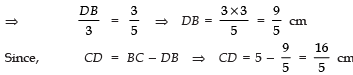Now, volume of the double cone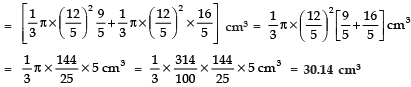Surface area of the double cone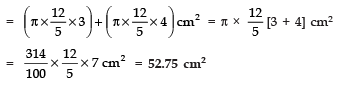Q 3. A cistern, internally measuring 150 cm × 120 cm × 110 cm, has 129600 cm3 of water in it. Porous bricks are placed in the water until the cistern is full to the brim. Each brick absorbs one-seventeenth of its own volume of water. How many bricks can be put in without overflowing the water, each being 22.5 cm × 7.5 cm × 6.5 cm?
Sol. ∵ Dimensions of the cistern are 150 cm, 120 cm and 110 cm.
∴ Volume of the cistern = 150 × 120 × 110 cm3 = 1980000 cm3
Volume of water contained in the cistern = 129600 cm3
∴ Free space (volume) which is not filled with water = 1980000 − 129600 cm3 = 1850400 cm3
Now, Volume of one brick = 22.5 × 7.5 × 6.5 cm3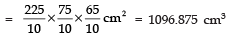∴ Volume of water absorbed by one brick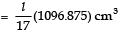Let ‘n’ bricks can be put in the cistern.
∴ Volume of water absorbed by ‘n’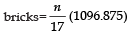∴ [Volume occupied by ‘n’ bricks] = [(free space in the cistern) + (volume of water absorbed by n-bricks)]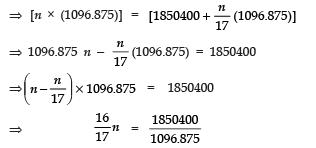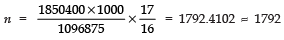Thus, 1792 bricks can be put in the cistern.

Q 4. In one fortnight of a given month, there was a rainfall of 10 cm in a river valley. If the area of the valley is 7280 km3, show that the total rainfall was approximately equivalent to the addition to the normal water of three rivers each 1072 km long, 75 m wide and 3 m deep.
Sol. Volume of three rivers = 3 {(Surface area of a river) × Depth}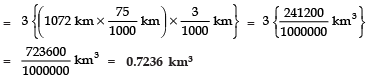Volume of rainfall = (surface area) × (height of rainfall)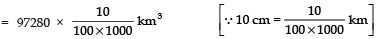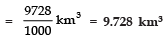Since, 0.7236 km3 ≠ 9.728 km3
∴ The additional water in the three rivers is not equivalent to the rainfall.

Q 5. An oil funnel made of tin sheet consists of a 10 cm long cylindrical portion attached to a frustum of a cone. If the total height is 22 cm, diameter of the cylindrical portion is 8 cm and the diameter of the top of the funnel is 18 cm, find the area of the tin sheet required to make the funnel (see Fig.).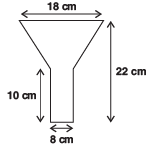Sol. We have, for the cylindrical part
Diameter = 8 cm
⇒ Radius (r) = 4 cm
Height = 10 cm
⇒ Curved Surface area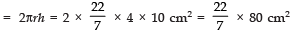For the frustum: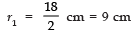and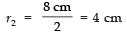Height (H) = 22 − 10 = 12 cm
∴ Slant height (l)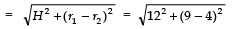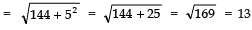∴Surface area,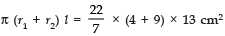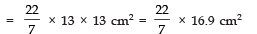Area of tin required = [Area of the frustum] + [Area of cylindrical portion]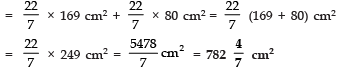Q 6. Derive the formula for the curved surface area and total surface area of the frustum of a cone, given to you in Section 13.5, using the symbols as explained.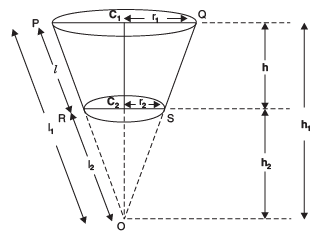Sol. We have,
Curved surface area of the frustum PQRS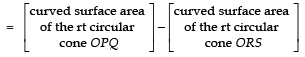= πr1 l1 − πr2 l2      ...(1)
Now,     ΔOC1 Q ~ ΔOC2S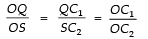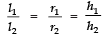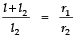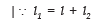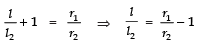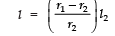Now, from (1), curved surface area of the frustum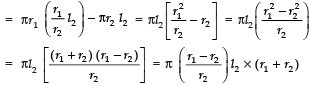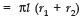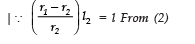Now, the total surface area of the frustum = (curved surface area) + (base surface area) + (top surfaces area)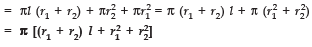Q 7. Derive the formula for the volume of the frustum of a cone, given to you in Section 13.5, using the symbols as explained.
Sol. We have, [Volume of the frustum RPQS]  = [Volume of right circular cone OPQ] − [Volume of right circular cone ORS]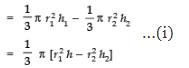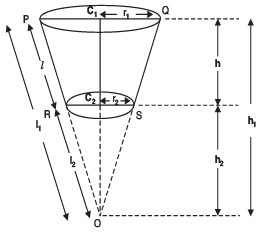Since ΔOC1Q ~ ΔOC2S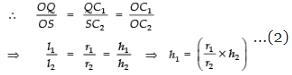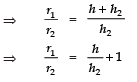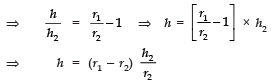From (1) and (2), we have
{volume of the frustum RPQS}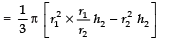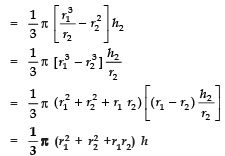Offer running on EduRev: Apply code STAYHOME200 to get INR 200 off on our premium plan EduRev Infinity!

,

,

,

,

,

,

,

,

,

,

,

,

,

,

,

,

,

,

,

,

,

;